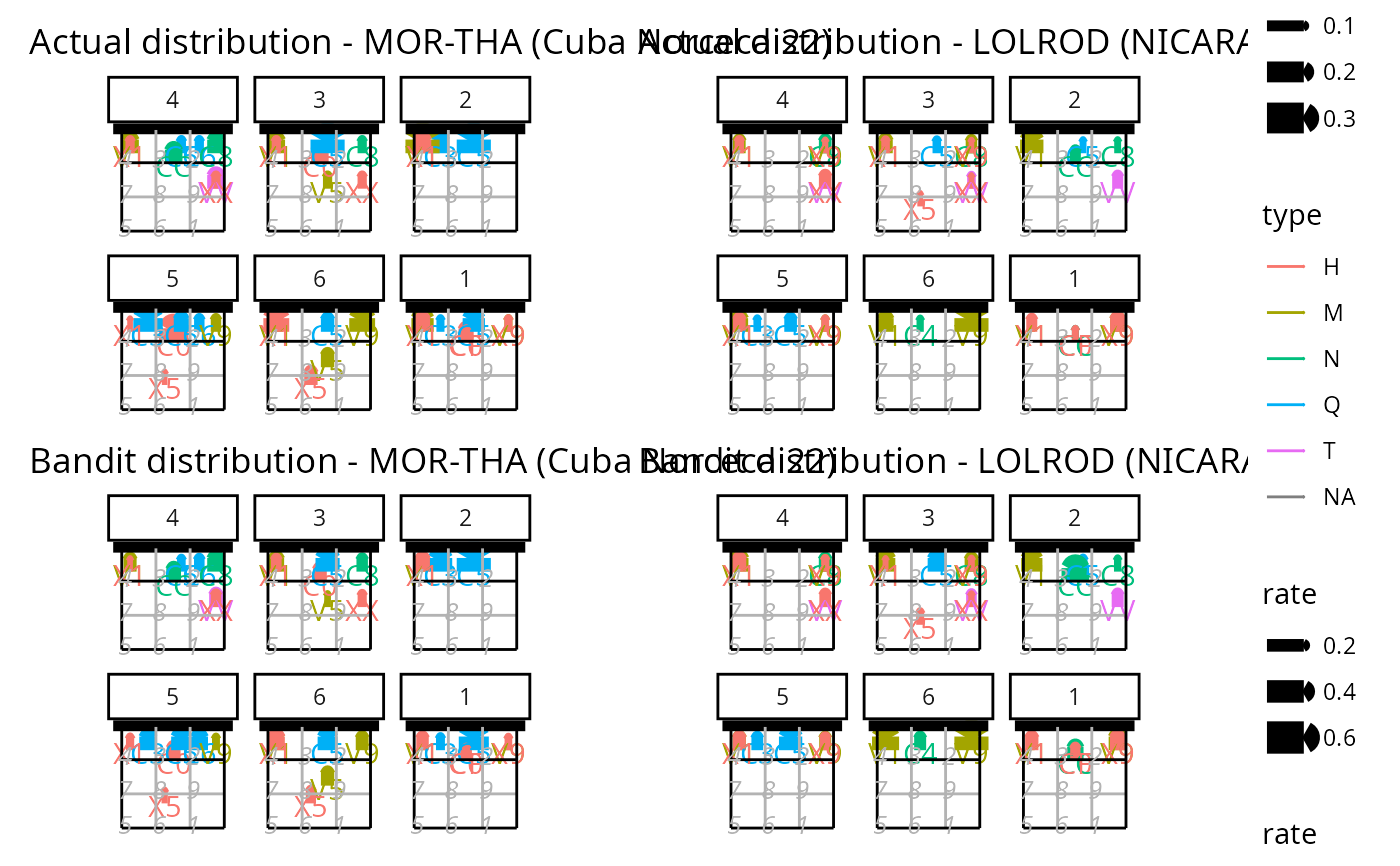Court plot of a real and simulated setter distribution

## Usage

ov_plot_distribution(
ssd,
label_setters_by = "id",
font_size = 11,
title_wrap = NA,
output = "plot"
)

## Arguments

ssd

simulated setter distribution output as returned by ov_simulate_setter_distribution()

label_setters_by

string: either "id" or "name"

font_size

numeric: font size

title_wrap

numeric: if non-NA, use strwrap() to break the title into lines of this width

output

string: either "plot" or "list"

## Examples

dvw <- ovdata_example("190301_kats_beds")
setter <- ov_simulate_setter_distribution(dvw = dvw, play_phase = c("Reception", "Transition"),
n_sim = 100, attack_by = "code")
ov_plot_distribution(setter)